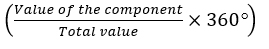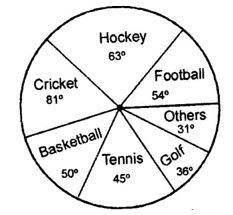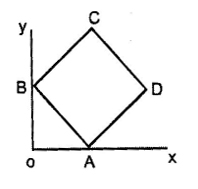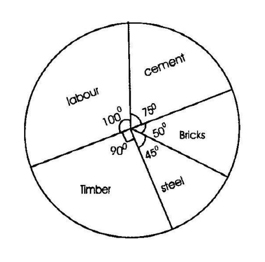# ICSE Worksheet for Chapter - 13 Graphs Class 8

## Find ICSE Worksheet for chapter-13 Graphs class 8

1. CLASS-8
2. BOARD: ICSE
3. Mathematic Worksheet - 13
4. TOPIC: Graphs
5. For other ICSE Worksheet for class 8 Mathematic check out main page of Physics Wallah.

#### SUMMARY

• The pie chart or a pie graph is a method of representing a given numerical data in the form of sectors of a circle.
• Central angle of the component =#### SECTION – I(OBJECTIVE)

1. Directions (Q.1 to 5): the circle graph given here shows the spending of a country on various sports during a particular year. Study the graph carefully and answer the questions given below it.
2.#### SECTION – II(SUBJECTIVE)

1. The number of students admitted in different faculties of a college are:
1. Faculty               Science            Arts         Commerce       Law         Education       Total
2. No. of students    1000              1200               650            450             300             3600
3. Construct the pie chart for the following distribution.
2. Given pie chart represents the expenditure on different items in constructing a flat in a metro city. Find the percentage expenditure on different items reading the pie chart, if the cost of the flat is Rs 5, 40,000. Find the following:1. i. The expenditure incurred on steel and cement separately.
2. ii. Difference of expenditures incurred on timber and bricks.

### Solutions: to worksheet-13 Topic- Graphs

1. A
2. D
3. C
4. B
5. A
6. B
7. D

#### Subjective:

1. 2) Rs 112500; Rs 6000